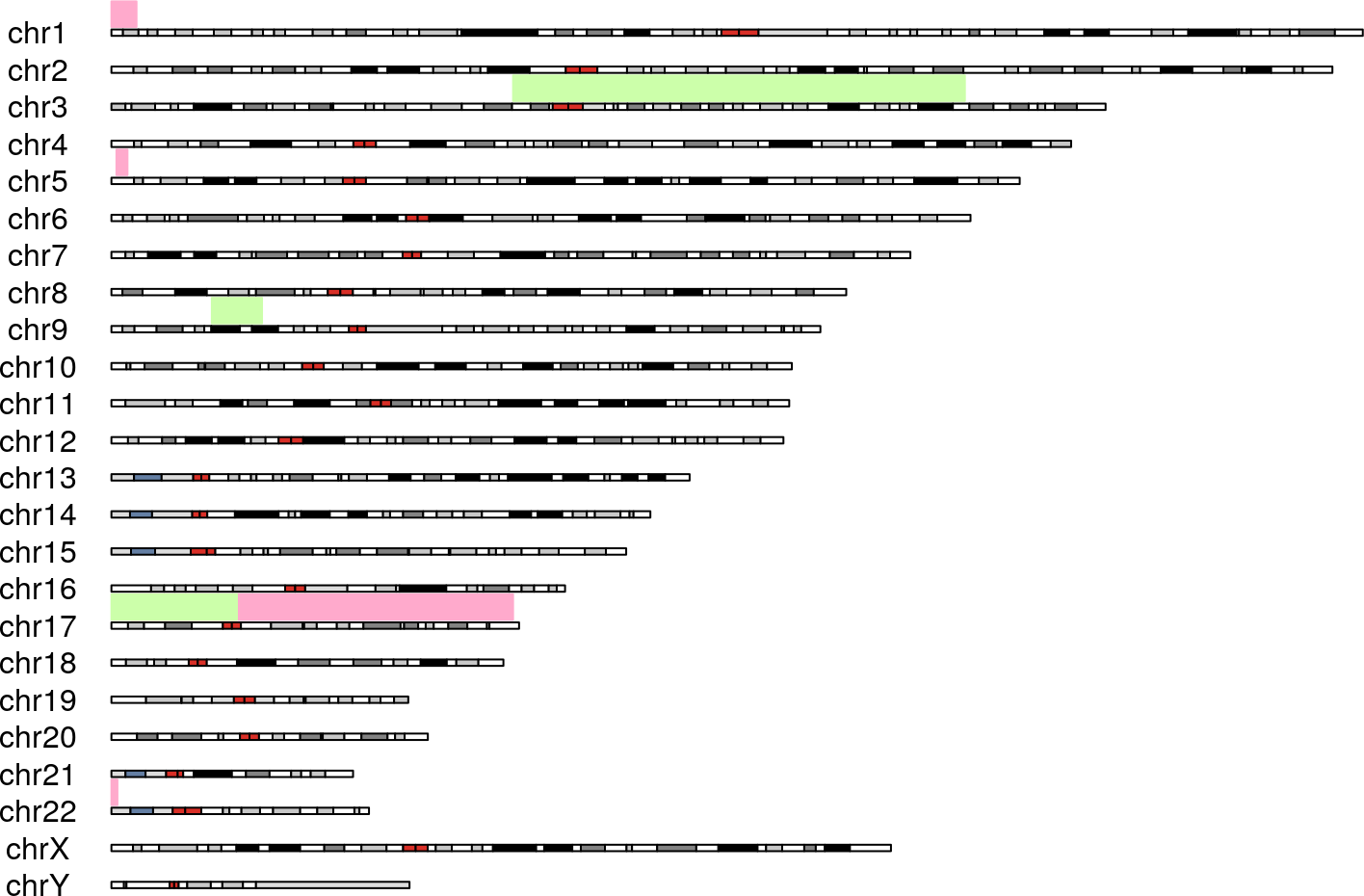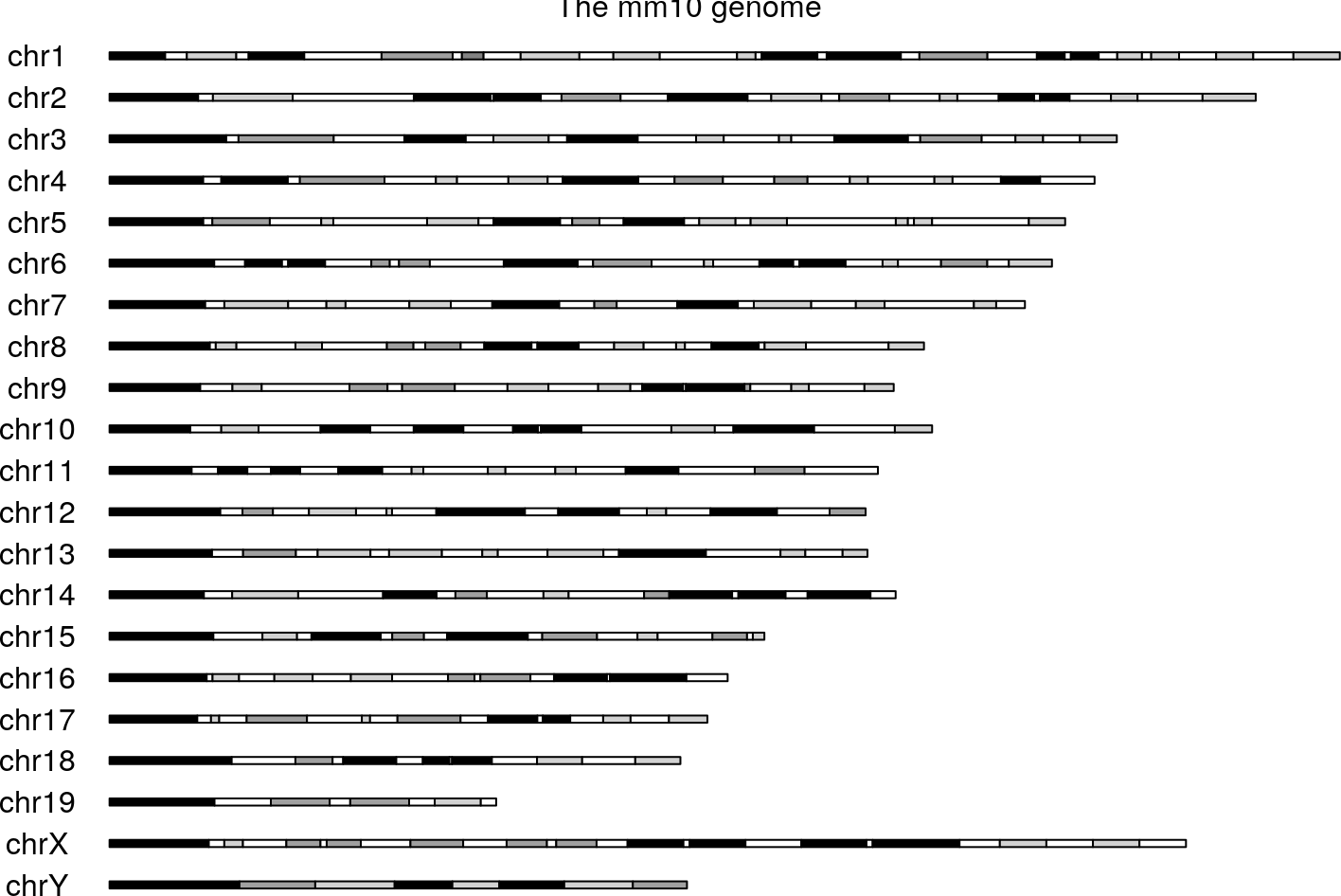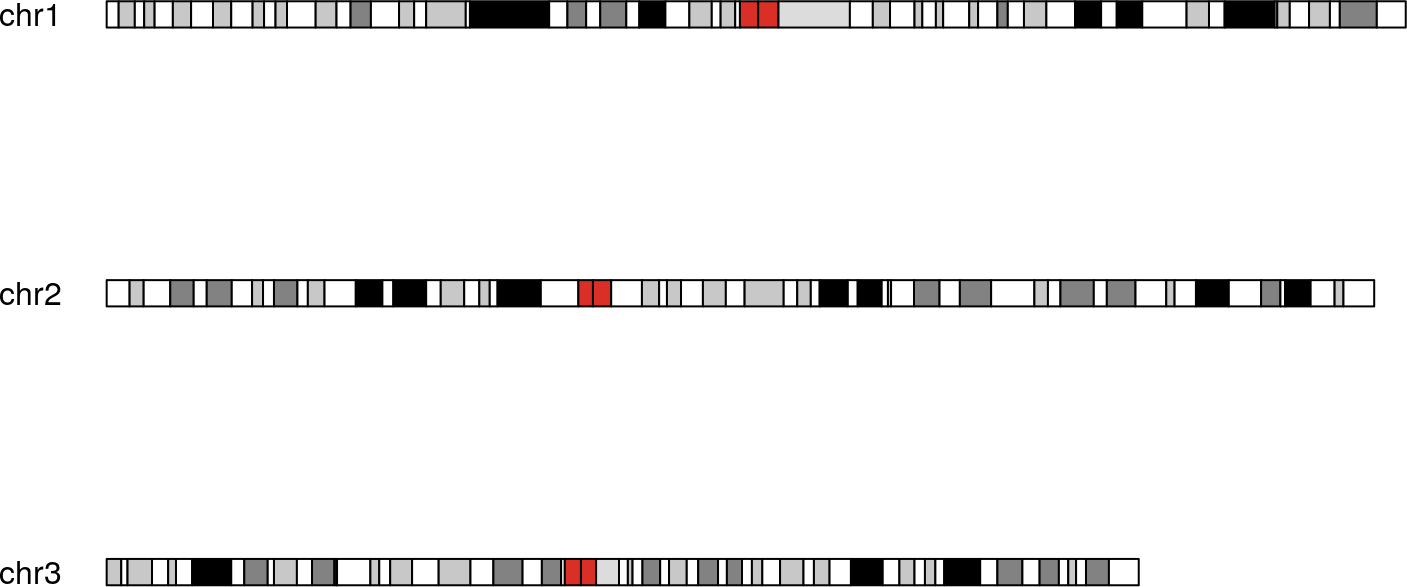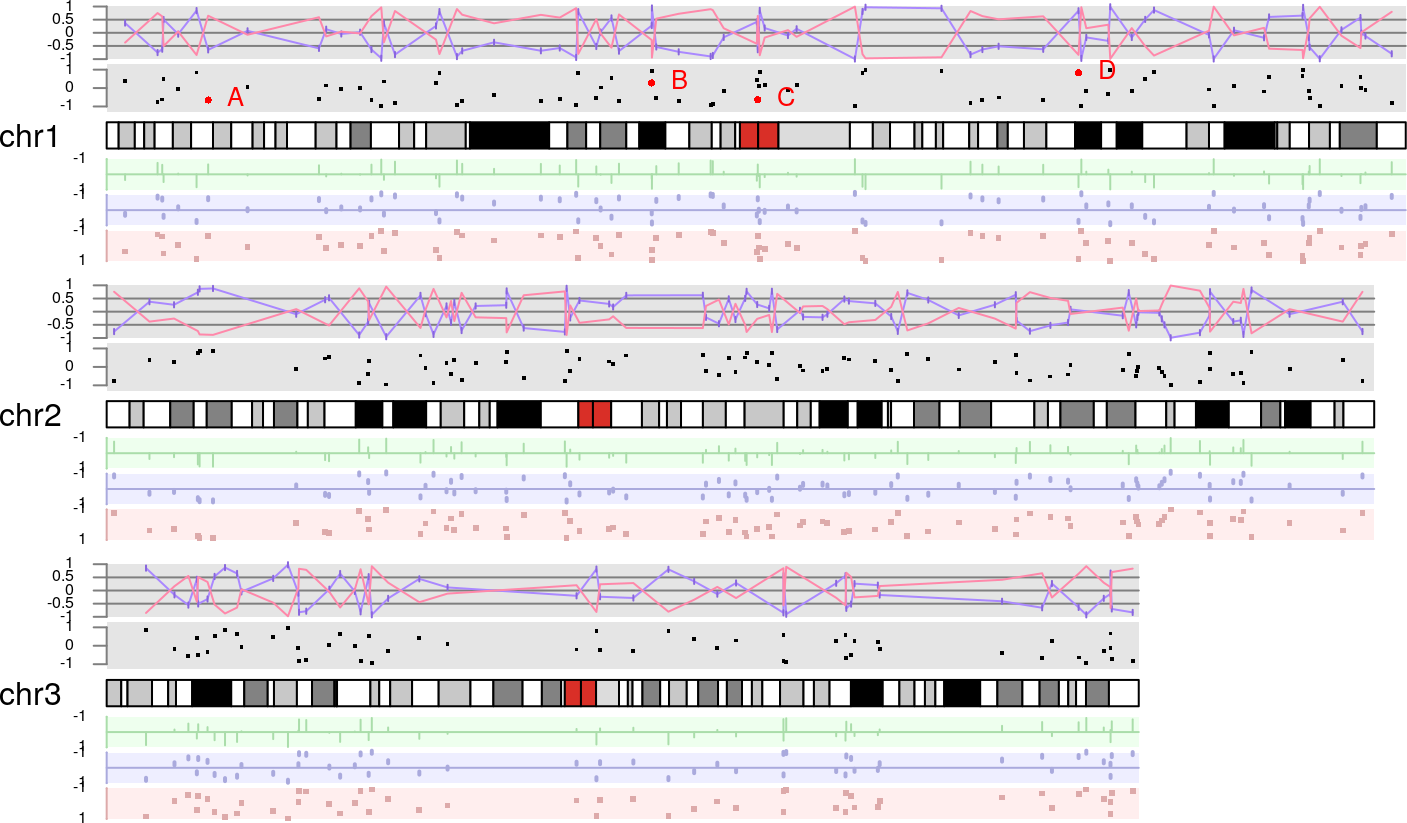# 1 Introduction

Data visualisation is a powerful tool used for data analysis and exploration in many fields. Genomics data analysis is one of these fields where good visualisation tools can be of great help. The aim of karyoploteR is to offer the user an easy way to plot data along the genome to get broad wide view where it is possible to identify genome wide relations and distributions.

karyoploteR is based on base R graphics and mimicks its interface. You first create a plot with `plotKaryotype` and then sequentially call a number of functions (`kpLines`, `kpPoints`, `kpBars`…) to add data to the plot.

karyoploteR is a plotting tool and only a plotting tool. That means that it is not able to download or retrieve any data. The downside of this is that the user is responsible of getting the data into R. The upside is that it is not tied to any data provider and thus can be used to plot genomic data coming from anywhere. The only exception to this are the ideograms cytobands, that by default are plotted using pre-downloaded data from UCSC.

# 2 Tutorial and Examples

In addition to this vignette, an detailed step-by-step tutorial and a set of complex examples with complete code are available at [https://bernatgel.github.io/karyoploter_tutorial/]

# 3 Quick Start

The basic idea behind karyoploteR has been to create a plotting system inspired by the R base graphics. Therefore, the basic workflow to create a karyoplot is to start with an empty plot with no data apart from the ideograms themselves using `plotKaryotype` and then add the data plots as required. To add the data there are functions based on the R base graphics low-level primitives -e.g `kpPoints`, `kpLines`, `kpSegments`, `kpRect`… - that can be used to plot virtually anything along the genome and other functions at a higher level useful to plot more specific genomic data types -e.g. `kpPlotRegions`, `kpPlotCoverage`…-.

This is a first simple example plotting a set of regions representing copy-number gains and losses using the `kpPlotRegions` function:

``````  gains <- toGRanges(data.frame(chr=c("chr1", "chr5", "chr17", "chr22"), start=c(1, 1000000, 4000000, 1),
end=c(5000000, 3200000, 80000000, 1200000)))
losses <- toGRanges(data.frame(chr=c("chr3", "chr9", "chr17"), start=c(80000000, 20000000, 1),
end=c(170000000, 30000000, 25000000)))
kp <- plotKaryotype(genome="hg19")
kpPlotRegions(kp, gains, col="#FFAACC")
kpPlotRegions(kp, losses, col="#CCFFAA")``````As you can see, the `plotKaryotype` returns a `KaryoPlot` object that has to be passed to any subsequent plot call.

`plotKaryotype` accepts a number of parameters but the most commonly used are `genome`, `chromosomes` and `plot.type`. The `genome` and `chromosomes` are used to specify the genome to be plotted (defaults to `hg19` and which chromosomes to plot (defaults to `canonical`). The `plot.type` parameter is used to select between different modes of adding data to the genome (above, below or on the ideograms).

For example, to create a plot of the mouse genome with data above the ideograms we would use this:

``  kp <- plotKaryotype(genome="mm10", plot.type=1, main="The mm10 genome")``And to plot the first thee chromosomes of the hg19 human genome assembly with data above and below them:

``  kp <- plotKaryotype(genome="hg19", plot.type=2, chromosomes=c("chr1", "chr2", "chr3"))``All low-level plotting functions share a similar interface, and in general, they accept the standard R plotting parameters (`lwd`, `cex`, `pch`, etc…). The simplest way (althought not always the most convenient) is to treat them as the equivalent R base plotting functions with an additional `chr` parameter. As an example, we can create a set of random 1 base regions (using regioneR `createRandomRegions`) and add a random `y` value to them:

``````  rand.data <- createRandomRegions(genome="hg19", nregions=1000, length.mean=1, length.sd=0,
``````##
## Attaching package: 'Biostrings'``````
``````## The following object is masked from 'package:base':
##
##     strsplit``````
``````  rand.data <- toDataframe(sort(rand.data))
rand.data <- cbind(rand.data, y=runif(n=1000, min=-1, max=1))

#Select some data points as "special ones"
sel.data <- rand.data[c(7, 30, 38, 52),]
``````##    chr    start      end           y
## 1 chr1  3534489  3534489  0.37182379
## 2 chr1  9765550  9765550 -0.75039143
## 3 chr1 10660469 10660469 -0.62290400
## 4 chr1 10918836 10918836  0.50535078
## 5 chr1 13722678 13722678 -0.05097132
## 6 chr1 17247042 17247042  0.84357538``````

And then plot them in different ways.

``````  kp <- plotKaryotype(genome="hg19", plot.type=2, chromosomes=c("chr1", "chr2", "chr3"))

kpDataBackground(kp, data.panel = 1, r0=0, r1=0.45)
kpAxis(kp, ymin=-1, ymax=1, r0=0.05, r1=0.4, col="gray50", cex=0.5)
kpPoints(kp, chr=rand.data\$chr, x=rand.data\$start, y=rand.data\$y,
ymin=-1, ymax=1, r0=0.05, r1=0.4, col="black", pch=".", cex=2)
kpPoints(kp, chr=sel.data\$chr, x=sel.data\$start, y=sel.data\$y,
ymin=-1, ymax=1, r0=0.05, r1=0.4, col="red")
kpText(kp, chr=sel.data\$chr, x=sel.data\$start, y=sel.data\$y,
ymin=-1, ymax=1, r0=0.05, r1=0.4, labels=c("A", "B", "C", "D"), col="red",
pos=4, cex=0.8)

#Upper part: data.panel=1
kpDataBackground(kp, data.panel = 1, r0=0.5, r1=1)
kpAxis(kp, ymin=-1, ymax=1, r0=0.5, r1=1, col="gray50", cex=0.5, numticks = 5)
kpAbline(kp, h=c(-0.5, 0, 0.5), col="gray50", ymin=-1, ymax=1, r0=0.5, r1=1)
kpLines(kp, chr=rand.data\$chr, x=rand.data\$start, y=rand.data\$y,
col="#AA88FF", ymin=-1, ymax=1, r0=0.5, r1=1)
#Use kpSegments to add small tic to the line
kpSegments(kp, chr=rand.data\$chr, x0=rand.data\$start, x1=rand.data\$start,
y0=rand.data\$y-0.1, y1=rand.data\$y+0.1,
col="#8866DD", ymin=-1, ymax=1, r0=0.5, r1=1)
#Plot the same line but inverting the data by pssing a r0>r1
kpLines(kp, chr=rand.data\$chr, x=rand.data\$start, y=rand.data\$y,
col="#FF88AA", ymin=-1, ymax=1, r0=1, r1=0.5)

#Lower part: data.panel=2
kpDataBackground(kp, r0=0, r1=0.29, color = "#EEFFEE", data.panel = 2)
kpAxis(kp, col="#AADDAA", ymin=-1, ymax=1, r0=0, r1=0.29, data.panel = 2,
numticks = 2, cex=0.5, tick.len = 0)
kpAbline(kp, h=0, col="#AADDAA", ymin=-1, ymax=1, r0=0, r1=0.29, data.panel = 2)
kpBars(kp, chr=rand.data\$chr, x0=rand.data\$start, x1=rand.data\$end, y1 = rand.data\$y,

kpDataBackground(kp, r0=0.34, r1=0.63, color = "#EEEEFF", data.panel = 2)
kpAxis(kp, col="#AAAADD", ymin=-1, ymax=1, r0=0.34, r1=0.63, data.panel = 2,
numticks = 2, cex=0.5, tick.len = 0)
kpAbline(kp, h=0, col="#AAAADD", ymin=-1, ymax=1, r0=0.34, r1=0.63, data.panel = 2)
kpSegments(kp, chr=rand.data\$chr, x0=rand.data\$start, x1=rand.data\$end,
y0=rand.data\$y-0.2, y1=rand.data\$y,
col="#AAAADD", ymin=-1, ymax=1, r0=0.34, r1=0.63, data.panel = 2, lwd=2)

kpDataBackground(kp, r0=0.68, r1=0.97, color = "#FFEEEE", data.panel = 2)
kpAxis(kp, col="#DDAAAA", ymin=-1, ymax=1, r0=0.68, r1=0.97, data.panel = 2,
numticks = 2, cex=0.5, tick.len = 0)
kpPoints(kp, chr=rand.data\$chr, x=rand.data\$start, y=rand.data\$y,
col="#DDAAAA", ymin=-1, ymax=1, r0=0.68, r1=0.97, data.panel = 2, pch=".", cex=3)``````The interface for the higher level plotting functions is a little different and they usually take Bioconductor objects (`GRanges`, etc…). As an example of these plotting functions we can create and plot 10 sets of 2000 random regions and see their coverage on the genome. In addition, we will plot the masked regions of the genomes where no random region should be.

``````  n <- 10 #number of sets of random regions
#Create random regions
all.regions <- lapply(seq_len(n), function(i) {
filterChromosomes(createRandomRegions(nregions=2000, length.mean=100000))
})
#join all regions into a single GRanges for coverage plotting
joined.regions <- do.call(c, all.regions)

#Create the plot
kp <- plotKaryotype(plot.type=2)